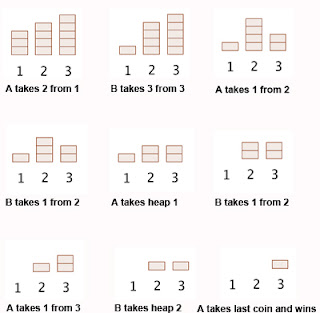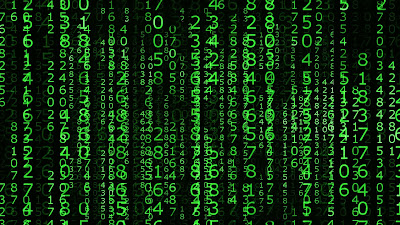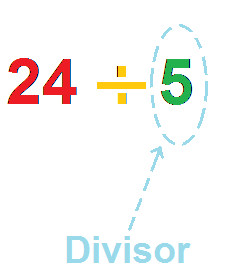## Friday, 26 August 2016## Colored Balls

### Introduction

Hello all, all of us have curiosities and we look on internet to find informations about it. In last time I just reflected over this topic. Today I am gonna show you aa new problem about Mr. Algorithm and a game with black and white balls.
If you know to solve this exercise and you think someone does not, then I invite you to be the first who give me the correct answer!

### Exercise

Mr. Algorithm has many balls of white and black colors. One day, he was playing with them. During the play, he arranged the balls into two rows both consisting of N number of balls. These two rows of balls are give to you in the form of strings X, Y; Both these strings consist of 'W' and 'B', where 'W' denotes a white colored ball and 'B' a black colored.
Other than these two rows of balls, Mr. Algorithm has an inifinite supply of extra balls of each color. He wants to create another row of N balls, Z in such a way that the sum of hamming distance between X and Z, and hamming distance between Y and Z is maximized.
Hamming Distance between two strings X and Y is defined as the number of positions where the color of balls in row X differs from the row Y ball at that position (e.g. hamming distance between "WBB", "BWB" is 2, as at position 1 and 2, corresponding colors in the two strings differ).
As there can be multiple such arrangements of row Z, Mr. Algorithm wants to find the lexicographically smalles arrangement which will maximize the above value.

Input:
The first line of the input contains an integer T denoting the number of test cases. The description of T test cases follows:
- first line of each test will contain a string X denoting the arrangement of balls in first row.
- second line will contain the string Y denoting the arrangement of balls in second row.

Output:
For each test case, output a single line containing the string of length N denoting the arrangement of colors of the balls belonging to row Z.
Constraints:
1 <= T <= 3
1 <= N <= 10^5

Example:
Input:
1
WBWB
WBBB

Output:
BWBW

### Conclusion

You will learn the following in this exercise:
- string manipulation
- game theory
- probabilities

If you don't know to solve this exercise, you can buy my solution for just \$1 (check bellow PayPal buttons):Join our community:
Google Plus

## Tuesday, 23 August 2016## Tweedle-Dee and Tweedle-Dum

"Tweedle-Dee and Tweedle-Dum are in a fierce battle playing even-odd nim. This novel game is played on N heaps. Heap i contains ai stones.
Like normal nim, Tweedle-Dee and Tweedle-Dum alternate taking a positive number of stones from any single one of the heaps, and the player that can't remove stones loses. However Tweedle-Dee can only take an even number of stones, and Tweedle-Dum can only take an odd number of stones.
Alice doesn't want to wait until the end of the game, so she asks you to determine the winner of the game. Remember that Tweedle-Dee and Tweedle-Dum are legendary grandmasters of combinatorial games, so they always play optimally."

### Input

The first line of the input contains an integer T denoting the number of test cases. The description of T test cases follows.
The first line of each case consists of an integer N the number of heaps, and a string P the player that starts the game. String P is equal to "Dee" if Tweedle-Dee goes first, or "Dum" if Tweedle-Dum goes first.
The second line of each case contains N space separated integers ai the number of stones of the i-th heap.

### Output

For each test case, output a single line containing either "Dee" if Twedle-Dee winns the game or "Dum" otherwise.

• 1T50
• 1N50
• 1ai50

### Example

```Input:
1
2 Dee
2 2

Output:
Dum ```
` `
`IfIf you would like to see my solution check below:`
`1. Without explanation: `2. With explanation:## Monday, 22 August 2016## Begginer Problem - Sticks

Hello all, after a long vacation, I come back with some new problems and new algorithms. Here is one for begginers and not only:

"Mr. Algorithm and his little brother are playing with sticks. They have total N sticks. Length of i-th stick is Ai. Mr. Algorithm asks his brother to choose any four sticks and to make a rectangle with those sticks its sides. Mr. Algorithm warns his brother to not to break any of the sticks, he has to use sticks as a whole. Also, he wants that the rectangle formed should have the maximum possible area among all the rectangles that Mr. Algorithm's brother can make. Mr. Algorithm's little brother takes this challenge up and overcomes it. Can you also do so? That is, you have to tell whether it is even possible to create a rectangle? If yes, then you have to tell the maximum possible area of rectangle."

Input: The first line contains a single integer T denoting the number of test-cases. T test cases follow. The first line of each test case contains a single integer N denoting the number of sticks. The second line of each test case contains N space-separated integers A1, A2, ..., AN denoting the lengths of sticks.

Output: For each test case, output a single line containing an integer representing the maximum possible area for rectangle or -1 if it's impossible to form any rectangle using the available sticks.

Constraints:
1 ≤ T ≤ 100
1 ≤ N ≤ 103
1 ≤ sum of N's over all test-cases in a single test file ≤ 103
1 ≤ Ai ≤ 103

Example:
Input:
2
5
1 2 3 1 2
4
1 2 2 3

Output:
2 -1

If you want, you can check my solution below for just 1 euro:## Sunday, 22 May 2016## Challenge #3 - Diagonal Difference

Given a square matrix of size , calculate the absolute difference between the sums of its diagonals.

Input Format:
The first line contains a single integer, . The next lines denote the matrix's rows, with each line containing space-separated integers describing the columns.
Output Format:
Print the absolute difference between the two sums of the matrix's diagonals as a single integer.

Sample Input: 3 11 2 4 4 5 6 10 8 -12
Sample Output: 15

What you will learn:
- get diagonals of a matrix

Check my optimized solution bellow (PayPal button):## Friday, 20 May 2016## Challenge #2

Mr. Algorithm have an integer number, which he want to reverse by following steps:
1. Convert the number into binary string.
2. Reverse binary string.
3. Convert the reversed binary string back to integer.
Can you help him write a function to do it ?

Example:
For `x = 234`, the output should be
`ReverseBit(x) = 87`.
`23410 = 111010102 => 010101112 = 8710`.
Input/Output
• [input] integer x
A non-negative integer.
• [output] integer
`x` reversed as described above.

If you want, you can check my solution for only 1 euro! (check PayPal button below):## Thursday, 19 May 2016## Number of divisors C++

Hello again. Here is another optimized algorithm to get number of divisors of a number! Check bellow button (PayPal button), only 1 euro!

P.S: Explanation of algorithm is inside file!## Contact

If you have any request please email me at
thealgorithm7@gmail.com and I will reply you as fast as I can.

You can request exercises, projects etc. in C++ (mainly) or in another programming language!

## Thursday, 12 May 2016## Challenge #1 - Crossing the bridge at night (C++ implementation)

Statement:
Mr. Algorithm and his friends like to party but all the party spots are across the bridge from where Mr. Algorithm live. The bridge is quite old unsafe, so only two people at a time can cross it and they need a lamp to do so at night. Unfortunately, his group only has one lamp.
This particular night is very cold, so you want to cross the bridge as fast as possible. For the i-th person in your group you know the time ti it will take them to cross the bridge. When two people i and j cross the bridge, they walk at the speed of the slowest of them, so it would take max(ti, tj) to get other side.

Calculate the minimal time your group will need to cross the bridge.

Input:
1 <= number_of_Mr.Algorithm_friends(include_him) <= 100
0 <= speed_for_each_person[i] <= 10^9

Output:
The minimum time required to cross the bridge.

Test case #1:
Input: [1, 2, 5, 10]
Output: 17

Test case #2:
Input: [1, 2, 3, 4, 5]
Output: 16

Test case #3:
Input: [1, 2, 3]
Output: 6

Test case #4:
Input: 3858721
Output: 3858721

Test case #5:
Input: [9, 1, 8, 2, 7, 3, 6, 4, 5, 9, 1, 8, 2, 7, 3, 6, 4, 5, 9, 1, 8, 2, 7, 3, 6, 4, 5, 9, 1, 8, 2, 7, 3, 6, 4, 5, 9, 1, 8, 2, 7, 3, 6, 4, 5, 9, 1, 8, 2, 7, 3, 6, 4, 5, 9, 1, 8, 2, 7, 3, 6, 4, 5, 9, 1, 8, 2, 7, 3, 6, 4, 5]
Ouput: 285

Test case #6:
Input: [1001, 2000, 2005, 2006]
Output: 8013

Test case #7:
Input: [1, 2, 10000, 0, 7]
Ouput: 10005

Test case #8:
Input: [239239239, 1, 239239239, 2]
Output: 239239246

HINT

Check the solution below(PayPal button):## Monday, 9 May 2016## Greatest common divisor

In mathematics, the greatest common divisor(gcd) of two or more integers, when at least one of them is not zero, is the largest positive integer that divides the numbers without a remainder(amount left over after peforming a division).

Example:
gcd(8, 12) = 4

The greatest common divisor is also known as the greatest common factor(gcf), highest common factor(hcf), greatest common measure(gcm) or highest common divisor.

I will present you the algorithm for this below:

#include <iostream>
using namespace std;

int gcd(int a, int b) // greatest common divisor of two numbers using repeated falls
{
while(a != b) // while this two numbers are different
{
if(a > b)
a = a - b;
else
b = b - a;
}
return a; // greatest common divisor will be saved in first number
}

int main()
{
cout<<gcd(8, 12);
cout<<endl;
return 0;
}
There are 5 more methods to find gcd, more optimized. One of them complexity is best. Check bellow buttons(PayPal)!!!Most optimized solution: